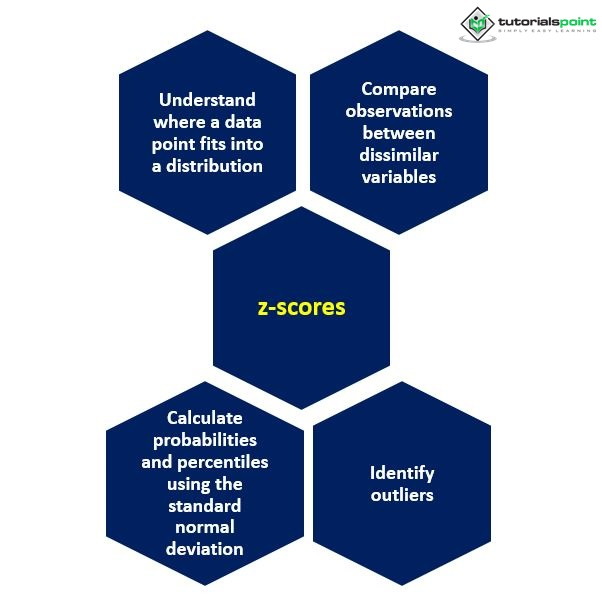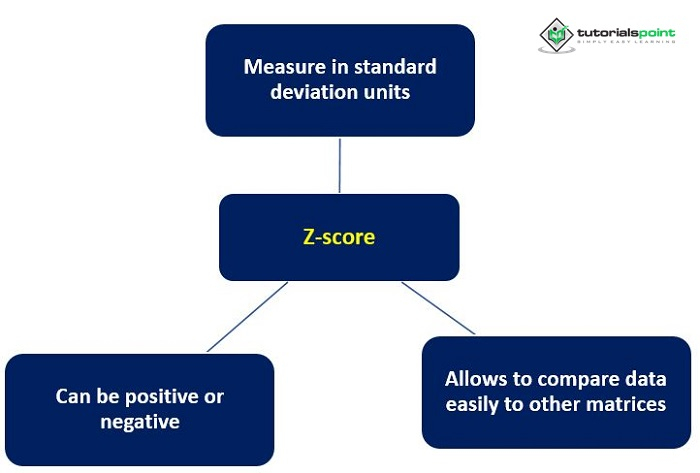# Application of Z-score in Psychology

For standard meaning, a common benchmark, and comparability, raw scores are frequently converted into standard scores. Such transformations can be linear or non-linear. The zero point of the scale and the unit of measurement for the scores are both subject to linear transformations. These alter the mean and standard deviation of the raw scores, but the differences between the transformed scores correspond closely to those between the respective raw scores in relative magnitude. So, the original shape, skewness, and kurtosis of the distribution of raw scores remain unaltered in that of the transformed scores.

## What is the Z-Score?

The simplest standard score is the linearly transformed z-score, standard deviation, or relative deviate. It measures the deviation of raw scores from the mean in terms of the standard deviation. The z-score expresses the deviation of a given raw score from the mean as many times the standard deviation. Irrespective of the original unit of raw scores, z-scores are expressed in standard deviation units or σ units. The z-score for the mean (µ) of the distribution amounts to zero. The advantages of standardizing the raw data by converting it into z-scores include the following

• Calculation of z-score

• Raw scores can be linearly transformed using the following equation## Calculation of z-score

Raw scores can be linearly transformed using the following equation

$\mathrm{X_s = \overline{X}_s +(X − \overline{X})\frac{s_s}{s}}$

Where,

$\mathrm{X_s}$ = transformed score

$\mathrm{\overline{X}_s}$ = mean of transformed score

$\mathrm{s_s}$ = standard deviation of transformed scores

$\mathrm{\overline{X}}$ = mean of raw score

s = standard deviation of raw score

On putting 0 as $\mathrm{\overline{x}}$, and 1 as $\mathrm{s_s}$, in the above-mentioned equation, the transformed score $\mathrm{X_s}$ is the z-score. Thus,

$\mathrm{z = \frac{X − \overline{X}}{s}}$

or,

$\mathrm{z = \frac{X − \mu}{\sigma}}$

Where, $\mathrm{x}$ = the value for which the z-score is calculated, also known as the raw score

$\mathrm{\mu}$ = the population mean

$\mathrm{\sigma}$ = the population standard deviation

The frequency distribution of a sample's z-scores, obtained through linear transformation, is identical to the original raw scores aside from the change in the unit to σ units and the modified zero point of the scale. The mean of the z-score distribution amounts to zero and is identical to the z-score for $\mu$, while the standard deviation of the distribution amount to one. So, z-scores are eminently suitable for comparing the scores of the same or different units or with widely different means and standard deviations.

Like the raw scores, the sample mean may be similarly transformed into the z-score, using the standard error (SE) of the mean $\mathrm{s_\overline{x}}$.

$\mathrm{z = \frac{\overline{X}-\mu}{\sigma_\overline{x}}}$

or,

$\mathrm{z = \frac{\overline{X}-\mu}{s_\overline{x}}}$

The z-score so computed expresses the deviation of the sample mean from the population mean in terms of the standard error. The z-score for $\mu$,

$\mathrm{z=\frac{(\mu-\mu)}{\sigma_\overline{x}}}$, amounts to $0$, around which the z-scores of the sample means to form a sampling distribution identical in shape and form a sampling distribution identical in shape and form with the sampling distribution of the corresponding sample means – it may be recalled that z-scores are linear transformed raw scores and consequently have the same form of the sampling distribution as those raw scores.

The z-score is also computed as the standard score to express the deviation of the difference (X1 − X2) between parametric means of the two populations from which the samples have been drawn. Where $\mathrm{s_{\overline{x}_{1} − \overline{x}_{2}}}$ is the estimated standard error of such difference between samples means,

$\mathrm{z = \frac{(\overline{X}_{1} − \overline{X}_{2}) − (\mu_1 − \mu_v)}{s_{\overline{x}_{1} − \overline{x}_{2}}}}$

Whenever the same population provides the source of both samples,

$\mathrm{\mu_1 − \mu_2 = 0}$

Therefore,

$\mathrm{z = \frac{\overline{X}_1 − \overline{X}_2}{s_{\overline{x}_1 − \overline{x}_2}}}$

## Interpretation of z-score

It includes:

### The z-scores are measured in standard deviation units

For example, a z-score of 1.2 shows that the observed value is 1.2 standard deviations from the mean. The closer z-score is to zero, the closer the value is to the mean. The value is further from the mean, the farther the z-score is from zero.

### The z-scores can be positive or negative

The z-scores for the mean of the distribution amount to zero, and it is positive for any score higher than the mean and negative for any lower than the mean. Thus, a raw score higher than the mean by an amount equal to 1.65 times the standard deviation has a z-score of +1.65 $\sigma$; a raw score lower than the mean by 1.96 times the standard deviation has a z-score of −1.96 $\sigma$.### The z-scores can quickly be compared to other metrics

Z-scores are useful for making comparisons between similar but different measurements. Height and weight, household income and debt levels, resting heart rates for men and women, and other parameters can all be compared using z-scores. The essential thing to remember is that the underlying distributions of the variables being compared should be comparable.

## Conclusion

The z-score for the difference between two samples means finds application in testing the significance of such a difference observed in an experiment. However, because the unit of z-scores is one σ which is relatively large, the computed z-score often has a value with a decimal.